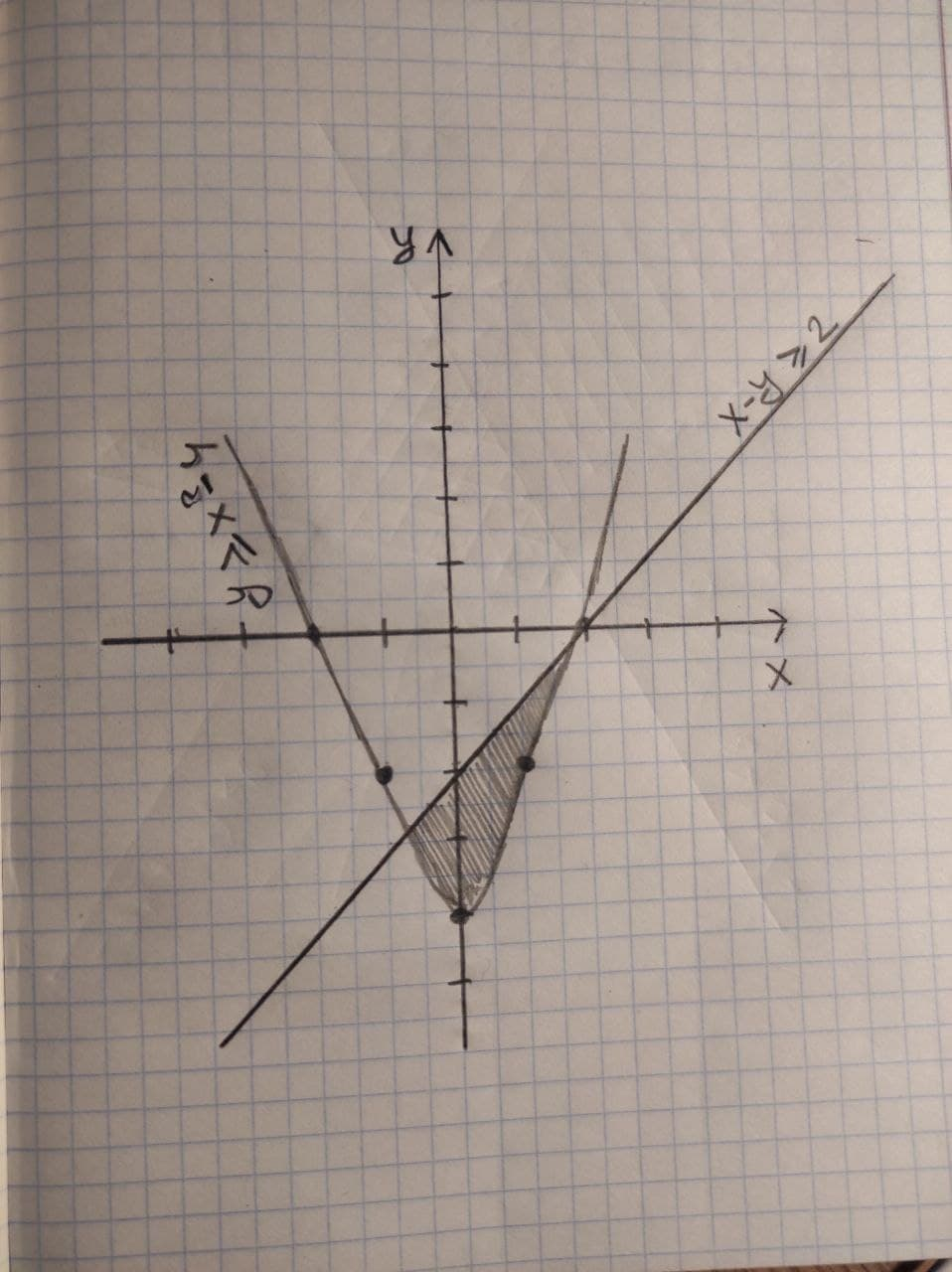# Graph the solution set of the system of inequalities or indicate that the system has no solution. {(y>=x^2-4),(x-y>=2):}Bevan Mcdonald 2020-12-30 Answered
Graph the solution set of the system of inequalities or indicate that the system has no solution.
$\left\{\begin{array}{c}y\ge {x}^{2}-4\\ x-y\ge 2\end{array}$
You can still ask an expert for help

## Want to know more about Inequalities systems and graphs?

• Questions are typically answered in as fast as 30 minutes

Solve your problem for the price of one coffee

• Math expert for every subject
• Pay only if we can solve itAyesha Gomez
The graph of shaded region of system of inequalities is: